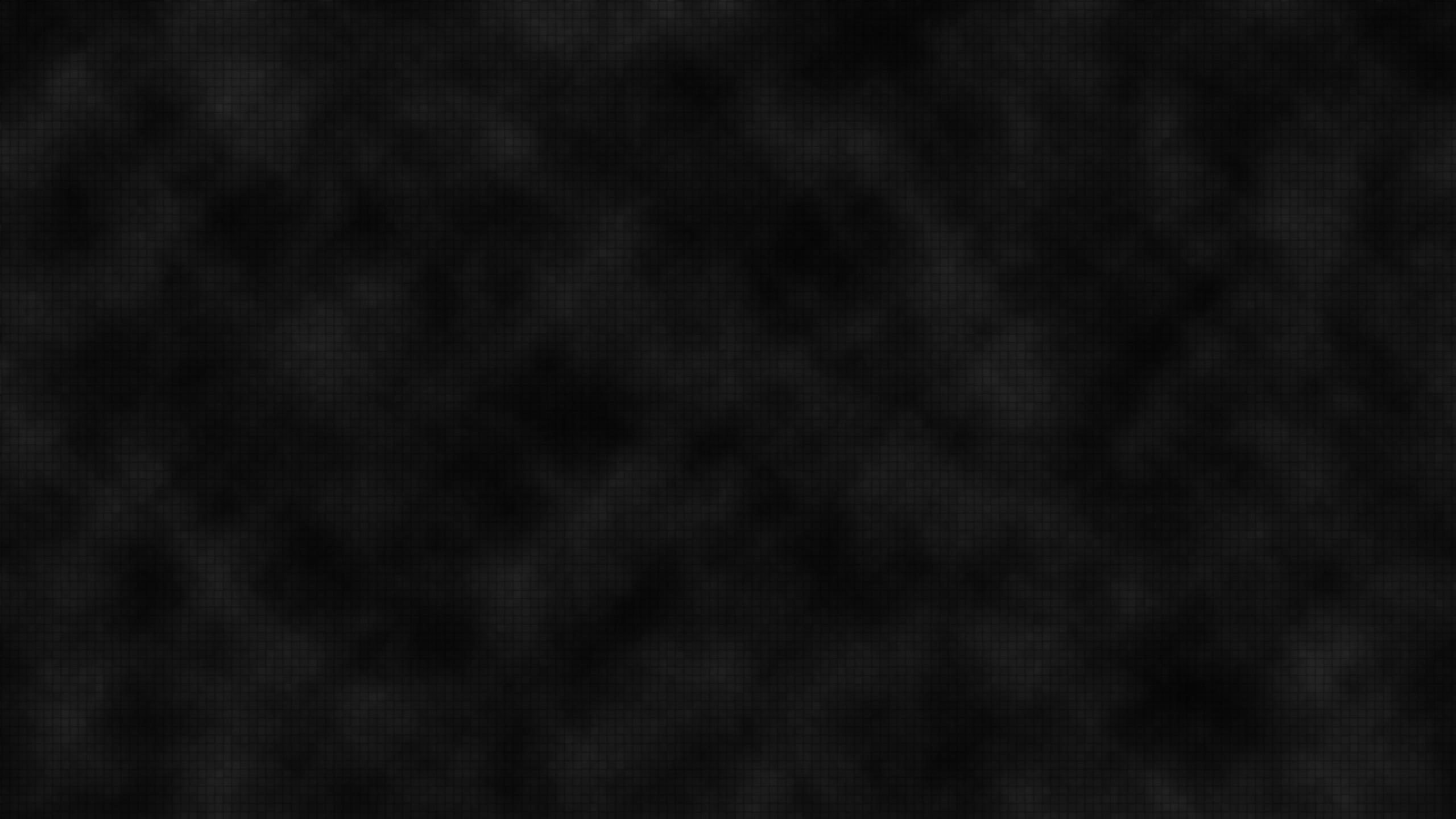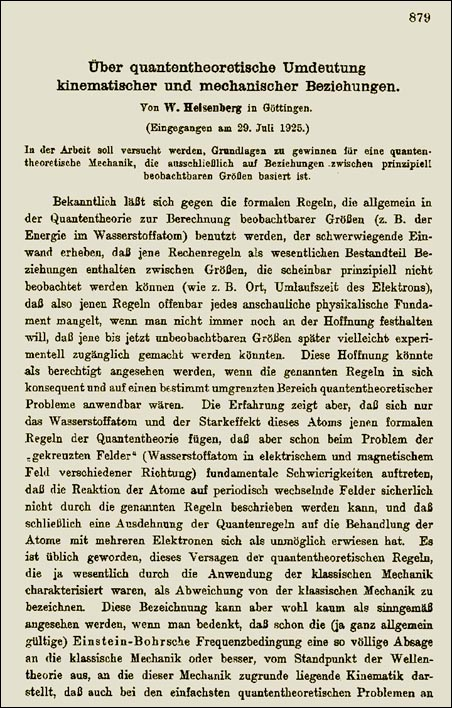Family Matters

1901-1910

High School Student

1911-1920

Youth Movement

1911-1920

University Student

1920-1927

Heisenberg's Doctorate

1920-1927

The Quantum Mechanic

1925-1927

The Uncertainty Principle

1925-1927

The Copenhagen Interpretation

1925-1927

Professor in Leipzig

1927-1942

Fission Research

1939-1945

Reviving German Science

1946-1976

Physics and Philosophy

1955-1956

A Brief Chronology

1901-1976

# Heisenberg's BreakthroughThe first page of Heisenberg's break-through paper on quantum mechanics, published in the Zeitschrift für Physik, 33 (1925), 879-893, received 29 July 1925. This image is reprinted from the facsimile reprint of the paper in Heisenberg, Collected Works/Gesammelte Werke, vol. A1, 382-396.

An English translation of the paper may be found in: B.L. Van Der Waerden, ed. and translator, Sources of Quantum Mechanics (New York: Dover, 1967), 261-276.

The basic idea of Heisenberg's paper was to get rid of the orbits in atoms and to arrive at new mechanical equations by working backwards from the observed frequencies and intensities of the light emitted and absorbed by matter. Working with an actual atom proved too complicated at this point. So Heisenberg studied instead a charged ball on a spring, an oscillator, whose motion was not quite regular (anharmonic).

Heisenberg looked first at the connection between the observable properties of the emitted light--its color (frequency) and the intensity--and the motion of the charged ball according to the classical mechanics of Newton. Then he considered the quantum properties of the observed light and reinterpreted the classical formulas for the motion in order to give the observed frequencies and intensities. This resulted in an unfamiliar rule for multiplying two amplitudes of the oscillation in order to obtain an intensity; normal multiplication gave the wrong result.

The unfamiliar rule may be expressed as follows. If two position variables can be expressed as Fourier series consisting of amplitudes A(n,k) and B(k,m), where n,m,k are integers, then multiplying two amplitudes together to obtain an intensity results in an infinite sum over all values of k:

### C(n,m) = Sk A(n,k) B (k,m)

This led to the puzzling result that the "commutation law" in arithmetic is no longer necessarily valid. That is, A times B does not necessarily equal B times A in quantum mechanics. This was particularly important when Heisenberg obtained the quantum mechanical expression corresponding to the "quantum conditions" in the old quantum theory. If the momentum p and the position q of a particle could be represented by Fourier series, then a differential expression for the multiplication pq in the old quantum theory became a difference expression in which pq does not equal qp. Instead, there is the famous "commutation relation" for the quantization condition that is at the basis of quantum mechanics:

### Sk p(n,k) q(k,n) - q(n,k) p(k,n) = h / 2pi, h being Planck's constant.

Born soon recognized Heisenberg's strange new multiplication rule as a rule that mathematicians had long used for the multiplication of special arrays of numbers--mathematical objects known as "matrices" (singular: matrix). Using the mathematics of matrices, scientists had at last a new mechanics for calculating the quantum behavior of particles, a quantum mechanics that was sometimes referred to as "matrix mechanics."